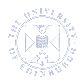# DEGREE REGULATIONS & PROGRAMMES OF STUDY 2015/2016University HomepageDRPS HomepageDRPS SearchDRPS Contact
DRPS : Course Catalogue : School of Physics and Astronomy : Undergraduate (School of Physics and Astronomy)

# Undergraduate Course: Methods of Theoretical Physics (PHYS10105)

 School School of Physics and Astronomy College College of Science and Engineering Credit level (Normal year taken) SCQF Level 10 (Year 3 Undergraduate) Availability Available to all students SCQF Credits 20 ECTS Credits 10 Summary The first part of the course, Complex Calculus, provides an introduction to complex numbers and analytic functions. We discuss the general properties of such functions, and develop the machinery of differential and integral calculus of complex functions. A special emphasis is put on using the Residue Theorem to evaluate real integrals. We introduce the Fourier and Laplace transforms and demonstrate how they can be used in solving linear PDE's. The second part of the course, Vectors, Tensors, and Continuum Mechanics, provides an introduction to continuum mechanics. It is used as a natural way to formally introduce the following concepts: vectors, bases, matrices determinants and the index notation, the general theory of Cartesian tensors, and rotation and reflection symmetries. These concepts are then applied in formulating a theory of elastic solids (linear elasticity) and viscous fluids (the Navier-Stokes equation) in terms of strain and stress tensors. These demonstrate how the resulting equations can be solved in various cases. We briefly introduce the concept of turbulence and describe its phenomenology and mechanism. Course description Complex calculus * Complex numbers review (operations, properties and inequalities) * Functions: a brief introduction to mapping. Limits & Continuity. Differentiation. Cauchy-Riemann relations * Analytic Functions: definition & properties. Introduction to conformal mapping. Branchs in � z, � z 2 � 1. * Dirichlet problem using conformal mapping * Complex integration: contours. Examples (1/z). Some theorems. * Antiderivatives. Complex fundamental theorem of calculus. Closed contours. * Cauchy-Goursat theorem. Deformation theorem. Applications of CauchyGoursat theorem: Cauchy's Integral Formula. Liouville's Theorem. Fundamental theorem of Algebra. Examples. * Morera's theorem. Complex series. Taylor series. Analytic continuation. * Laurent Series. Examples. Zeroes & Singularities. Residues. Examples. * Residue theorem. Jordan's Lemma, Indentation Lemma. Examples. * Branches and branch cuts. Improper integrals. Kramers-Kronig relations. * Fourier and Laplace transforms. Solving ODE's with Fourier and Laplace transforms. Vectors, Tensors, and Continuum Mechanics. * Vectors, bases, Einstein summation convention, the delta and epsilon symbols, matrices, determinants. * Rotations of bases, composition of two rotations, reflections, projection operators, passive and active transformations, the SO(3) symmetry group. * Cartesian tensors: - definition/transformation properties and rank - quotient theorem, pseudotensors, the delta and epsilon symbols as tensors - isotropic (pseudo)tensors * Taylor's theorem: the one- and three-dimensional cases * Linear Elasticity - the strain tensor, stretching and shear - the stress tensor, and some properties - elastic deformations of solid bodies, generalised Hooke's Law, isotropic media (and the various parameterisations for constants, ie Lam�e constants; Young's modulus and Poisson's ratio; bulk and shear modulus) * Fluid Mechanics - The Navier-Stokes equation. Incompressibility. The Reynolds number. Simple exact solutions. - Stokes equation. Drag on a sphere. Method of singularities. Multipole expansion. - Advection-diffusion equation. - Phenomenon of the transition to turbulence. Kolmogorov-Richardson cascade (qualitatively!). - Linear viscoelasticity
 Pre-requisites Students MUST have passed: Dynamics and Vector Calculus (PHYS08043) AND Fourier Analysis and Statistics (PHYS09055) OR Fourier Analysis (MATH10051) Co-requisites Prohibited Combinations Students MUST NOT also be taking Complex Analysis (PHYS10091) AND Symmetries of Classical Mechanics (PHYS10088) AND Electromagnetism and Relativity (PHYS10093) AND Honours Complex Variables (MATH10067) Other requirements None
 Pre-requisites None High Demand Course? Yes
 Academic year 2015/16, Available to all students (SV1) Quota:  None Course Start Semester 2 Timetable Timetable Learning and Teaching activities (Further Info) Total Hours: 200 ( Lecture Hours 44, Supervised Practical/Workshop/Studio Hours 40, Summative Assessment Hours 6, Programme Level Learning and Teaching Hours 4, Directed Learning and Independent Learning Hours 106 ) Assessment (Further Info) Written Exam 80 %, Coursework 20 %, Practical Exam 0 % Additional Information (Assessment) Coursework: 20% Examination: 80% Feedback Not entered Exam Information Exam Diet Paper Name Hours & Minutes Main Exam Diet S2 (April/May) 3:00
 On completion of this course, the student will be able to: State in precise terms the foundational principles of complex analysis and continuum mechanics and how they relate to broader physical principles.Devise and implement a systematic strategy for solving a complex problem in complex analysis or continuum mechanics by breaking it down into its constituent parts.Apply a wide range of mathematical techniques, such as Cauchy's Integral Formula, Residue Theorem, Fourier and Laplace transforms, solving ODEs and PDEs, asymptotic matching technique, etc., to build up the solution to a complex problem in theoretical physics.Use experience and intuition gained from solving physics problems to predict the likely range of reasonable solutions to an unseen problem.Resolve conceptual and technical difficulties by locating and integrating relevant information from a diverse range of sources.
 None
 Graduate Attributes and Skills Not entered Keywords MoTP
 Course organiser Dr Miguel Martinez-Canales Tel: (0131 6)51 7742 Email: miguel.martinez@ed.ac.uk Course secretary Mrs Siobhan Macinnes Tel: (0131 6)51 3448 Email: Siobhan.MacInnes@ed.ac.uk
 Navigation Help & Information Home Introduction Glossary Search DPTs and Courses Regulations Regulations Degree Programmes Introduction Browse DPTs Courses Introduction Humanities and Social Science Science and Engineering Medicine and Veterinary Medicine Other Information Combined Course Timetable Prospectuses Important Information
© Copyright 2015 The University of Edinburgh - 18 January 2016 4:44 am